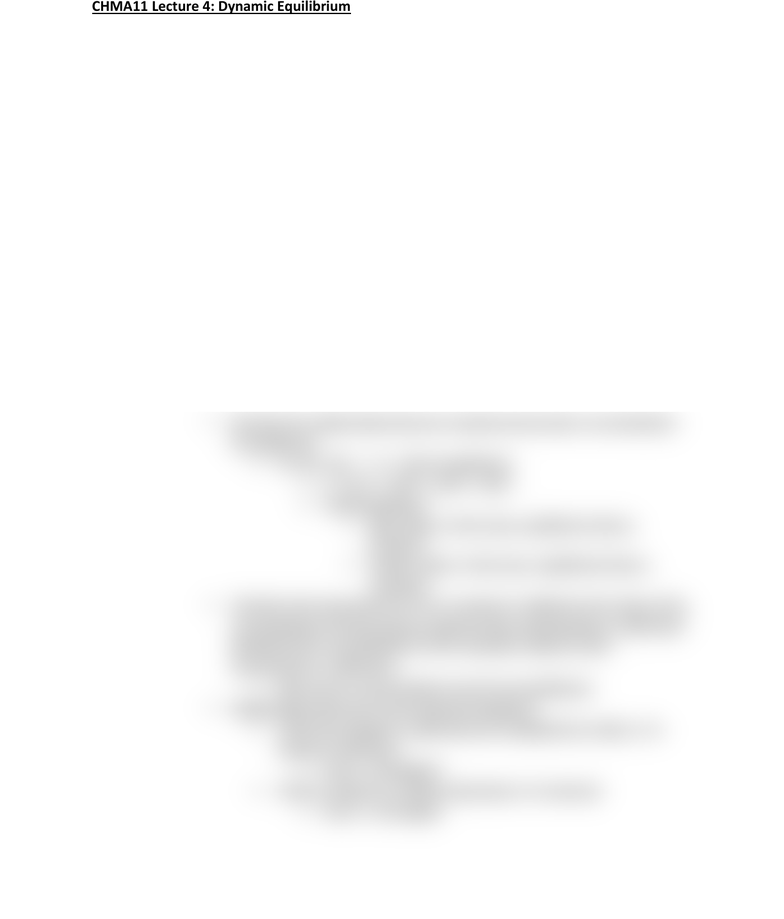# CHMA11H3 Lecture Notes - Lecture 4: Dynamic Equilibrium, Equilibrium Constant, Chemical Equation

110 views2 pages
School
Department
CourseCHMA11 Lecture 4: Dynamic Equilibrium
- Dynamic Equilibrium: point in time when the forward and reverse reaction rates are
equal for a given reaction
o Reversible Reactions: reactions able to proceed in both the forward and reverse
directions
o Dynamic equilibrium is called Dynamic due to the fact that both the forward and
reverse reactions are still occurring
They are occurring at the same rate
o No change in concentration in the products or reactants is seen once dynamic
equilibrium is reached
The concentrations of products and reactants doesn’t have to be the
same
Some reactions reach equilibrium after most of the reactant has
been consumed
o In these cases, equilibrium favors products
Some reactions reach equilibrium after most of the product has
been consumed
o In these cases, equilibrium favors reactants
o Equilibrium constant (K): allows us to quantify the relative amounts of reactants
and products at equilibrium
Denotes the relationship between reactant and product concentrations
at equilibrium
For aA + bB -> cC + dD at equilibrium
o K = [(C)c x (D)d ] / [(A)a x (B)b ]
o Understanding K
Big K value: in this case, equilibrium favors
products
Small K value: in this case, equilibrium favors
reactants
Therefore the expression for K for a reaction is defined as the ratio of the
concentrations of the products raised to their stoichiometric coefficients
divided by the concentrations of the reactants raided to their
stoichiometric coefficients
Both sets of concentrations must be at equilibrium
Relationships between K and chemical equations
When the equation coefficients are multiplied by a factor, K is
raised to that factor
o Knew = (Koriginal)n
When a reaction is written backwards, K in inversed
o Knew = 1/Koriginal
Unlock document

This preview shows half of the first page of the document.
Unlock all 2 pages and 3 million more documents.